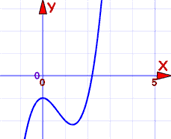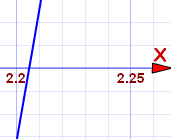# Approximate Solutions

Sometimes it is difficult to solve an equation exactly. But an approximate answer may be good enough!

## What is Good Enough?

Well, that depends what you are working on!

• If you are dealing with millions of dollars then you should try to get pretty close indeed. And that might need many significant digits.
• If you are calculating how much food to buy for a party, then a small error won't matter so much. You could always buy a little extra to be sure.
• Or something in between

So understanding what you are working on helps you know how accurate you should be.

## Solving Equations

To help reduce error, when solving equations:

• first solve for x = something
• then do any calculations

Like this:

### Example: Solve x/7 − 6.3068 + 2π = 0   (to 3 decimal places)

Start with:x/7 − 6.3068 + 2π = 0
Subtract −6.3068+2π from both sides:x/7 = +6.3068 − 2π
Multiply by 7:x = 7(6.3068 − 2π)
NOW do the calculations:x = 0.165

Why wait until the end to do the calculations? Well, every time you do a calculation you can introduce an error. If you do this several times your errors can accumulate to be quite large.

## Checking

If your answer is approximate, then your checking will also be approximate.

### Example: Check that x = 0.165 solves x/7 − 6.3068 + 2π = 0

Substitute 0.165 for x:0.165/7 − 6.3068+ 2π = 0
Calculate:−0.00004 = 0

Not quite right, but very close.

## Graphical Estimation

You can make good approximations using graphs, particularly by using a zoom function, like on our Function Grapher.

Here is an example:

### Example: estimate the solution to x3 − 2x2 − 1 = 0 (to 2 decimal places).

Solution: Plot it!

Here is my first attempt. I can see it crosses through y=0 at about x=2.2Let us zoom in there to see if we can see the crossing point better:It crosses between 2.20 and 2.21 ... slightly closer to 2.21. We are asked for 2 decimal places, so our answer is:

x3 − 2x2 − 1 = 0 at about x = 2.21

Check: (2.21)3 − 2(2.21)2 + 2 = approx 0.025, close to y=0